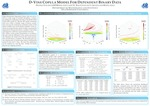## College of Sciences Posters

#### Title

D-Vine Copula Model For Dependent Binary Data

#### College

College of Sciences

#### Program

Ph.D. Computational and Applied Mathematics -- Mathematics and Statistics

Spring 2020

#### DOI

10.25883/wspk-kp13

#### Abstract

High-dimensional dependent binary data are prevalent in a wide range of scientific disciplines. A popular method for analyzing such data is the Multivariate Probit (MP) model. But the MP model sometimes fails even within a feasible range of binary correlations, because the underlying correlation matrix of the latent variables may not be positive definite. In this research, we proposed pair copula models, assuming the dependence between the binary variables is first order autoregressive (AR(1))or equicorrelated structure. Also, when Archimediean copula is used, most paper converted Kendall Tau to corresponding copula parameter, there is no explicit function of Pearson’s correlation coefficient with copula parameter. Therefore, we obtain the relationship between binary variable coefficient with copula parameter in the study as well. The outline of this poster presentation is as follows: we start with the definition of the copula and pictorially illustrate the relation between the copula parameter and the binary correlation. We illustrate pair copula constructions of multivariate binary distributions using D-vines and C-vines. We show the application of our method on a real life data. Finally, we briefly discuss our ongoing research.

#### Keywords

Binary data, Multivariate Probit (MP) model, D-vine, Copula parameter, Coefficient

#### Disciplines

Applied Statistics | Numerical Analysis and Computation | Probability

#### Files